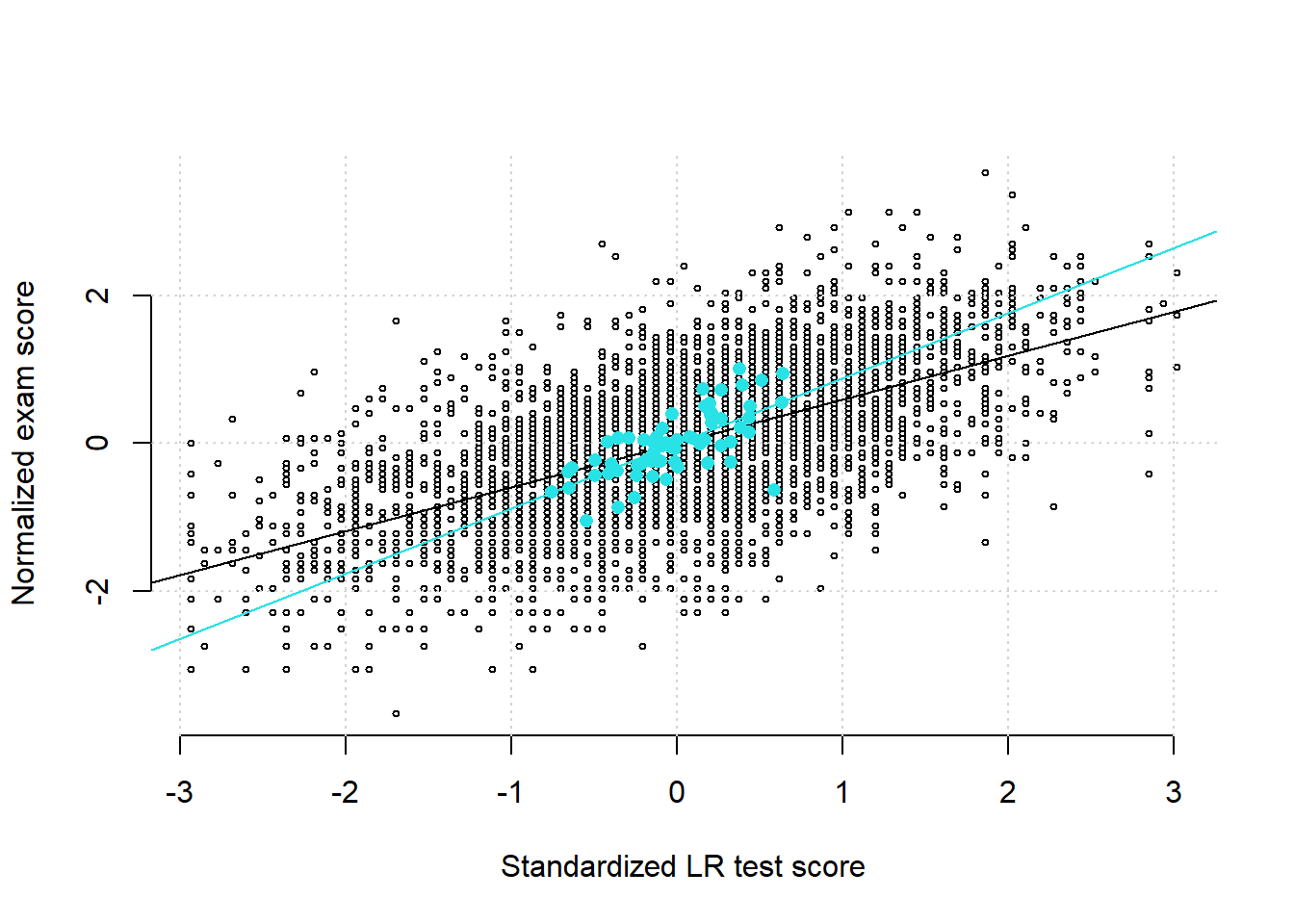## Problem statement

Draw a scatter plot of the variable normexam against the variable standLRT from the data set Exam{mlmRev}. Superimpose on it another scatter plot using the means of the same two variables by school. You can start by editing this rmarkdown template in RStudio before submitting the output to moodle.

## Data management

In this section, we load the exam scores data set, activate the help page for it, and examine the first 6 lines of the data frame object.

# install a contributed package
#install.packages("mlmRev")
# load the package to working directory
library(mlmRev)
# load the data from the package
data(Exam, package="mlmRev")
# invoke help document
?Exam
# view first 6 lines
head(Exam)
  school normexam schgend  schavg      vr     intake standLRT sex type student
1      1  0.26132   mixed 0.16618 mid 50% bottom 25%  0.61906   F  Mxd     143
2      1  0.13407   mixed 0.16618 mid 50%    mid 50%  0.20580   F  Mxd     145
3      1 -1.72388   mixed 0.16618 mid 50%    top 25% -1.36458   M  Mxd     142
4      1  0.96759   mixed 0.16618 mid 50%    mid 50%  0.20580   F  Mxd     141
5      1  0.54434   mixed 0.16618 mid 50%    mid 50%  0.37111   F  Mxd     138
6      1  1.73490   mixed 0.16618 mid 50% bottom 25%  2.18944   M  Mxd     155

## Summary statistics

We first compute the correlation coefficient between exam scores and LRT scores using all students in the data set. We then compute the mean exam scores and mean LRT scores by school. The correlation coefficient between mean school exam scores and mean school LRT scores is then calculated.

with(Exam, cor(normexam, standLRT))
 0.59165
# compute the means by school
mLRT <- with(Exam, tapply(standLRT, school, mean))
mexam <- with(Exam, tapply(normexam, school, mean))
cor(mexam, mLRT)
 0.69241

## Visualization

We draw a scatter diagram of the exam scores against the LRT scores and add the regression line. Next we superimpose on the scatter plot the mean school exam scores and mean school LRT scores (in color cyan) and add the regression line based on the mean school scores.

with(Exam, plot(normexam ~ standLRT,
bty = 'n',
cex = 0.5,
xlab = 'Standardized LR test score',
ylab = 'Normalized exam score'))
grid()
with(Exam, abline(lm(normexam ~ standLRT)))
points(mLRT, mexam, pch=16, col=5)
abline(lm(mexam ~ mLRT), col=5)